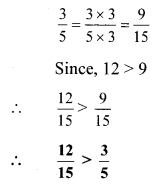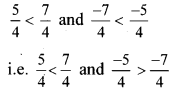# Maharashtra Board Class 8 Maths Solutions Chapter 1 Rational and Irrational Numbers Practice Set 1.2

## Maharashtra State Board Class 8 Maths Solutions Chapter 1 Rational and Irrational Numbers Practice Set 1.2

Question 1.
Compare the following numbers.
i. 7, -2
ii. 0, $$\frac { -9 }{ 5 }$$
iii. $$\frac { 8 }{ 7 }$$, 0
iv. $$-\frac{5}{4}, \frac{1}{4}$$
v. $$\frac{40}{29}, \frac{141}{29}$$
vi. $$-\frac{17}{20},-\frac{13}{20}$$
vii. $$\frac{15}{12}, \frac{7}{16}$$
viii. $$-\frac{25}{8},-\frac{9}{4}$$
ix. $$\frac{12}{15}, \frac{3}{5}$$
x. $$-\frac{7}{11},-\frac{3}{4}$$
Solution:
i. 7, -2
If a and b are positive numbers such that a < b, then -a > -b.
Since, 2 < 7 ∴ -2 > -7

ii. 0, $$\frac { -9 }{ 5 }$$
On a number line, $$\frac { -9 }{ 5 }$$ is to the left of zero.
∴ 0 > $$\frac { -9 }{ 5 }$$

iii. $$\frac { 8 }{ 7 }$$, 0
On a number line, zero is to the left of $$\frac { 8 }{ 7 }$$ .
∴ $$\frac { 8 }{ 7 }$$ > 0

iv. $$-\frac{5}{4}, \frac{1}{4}$$
We know that, a negative number is always less than a positive number.
∴ $$-\frac{5}{4}<\frac{1}{4}$$

v. $$\frac{40}{29}, \frac{141}{29}$$
Here, the denominators of the given numbers are the same.
Since, 40 < 141
∴ $$\frac{40}{29}<\frac{141}{29}$$

vi. $$-\frac{17}{20},-\frac{13}{20}$$
Here, the denominators of the given numbers are the same.
Since, 17 < 13
∴ -17 < -13
∴ $$-\frac{17}{20}<-\frac{13}{20}$$

vii. $$\frac{15}{12}, \frac{7}{16}$$
Here, the denominators of the given numbers are not the same.
LCM of 12 and 16 = 48Alternate method:
15 × 16 = 240
12 × 7 = 84
Since, 240 > 84
∴ 15 × 16 > 12 × 7viii. $$-\frac{25}{8},-\frac{9}{4}$$
Here, the denominators of the given numbers are not the same.
LCM of 8 and 4 = 8ix. $$\frac{12}{15}, \frac{3}{5}$$
Here, the denominators of the given numbers are not the same.
LCM of 15 and 5 = 15x. $$-\frac{7}{11},-\frac{3}{4}$$
Here, the denominators of the given numbers are not the same.
LCM of 11 and 4 = 44#### Maharashtra Board Class 8 Maths Solutions Chapter 1 Rational and Irrational Numbers Practice Set 1.2 Questions and Activities

Question 1.
Verify the following comparisons using a number line. (Textbook pg. no, .3)
i. 2 < 3 but – 2 > – 3
ii. $$\frac{5}{4}<\frac{7}{4}$$ but $$\frac{-5}{4}<\frac{-7}{4}$$
Solution:We know that, on a number line the number to the left is smaller than the other.
∴ 2 < 3 and -3 < -2
i.e. 2 < 3 and -2 > -3